# The SYSLIN Procedure

### Computational Details

Subsections:

This section discusses various computational details.

#### Computation of Least Squares-Based Estimators

Let the system be composed of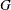equations and let the ith equation be expressed in this form: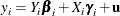whereis the vector of observations on the dependent variable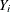is the matrix of observations on the endogenous variables included in the equation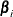is the vector of parameters associated with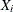is the matrix of observations on the predetermined variables included in the equation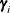is the vector of parameters associated withis a vector of errors

Let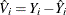, where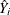is the projection ofonto the space spanned by the instruments matrix Z.

Let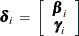be the vector of parameters associated with both the endogenous and exogenous variables.

The K-class of estimators (Theil, 1971) is defined by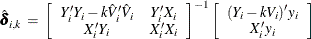where k is a user-defined value.

Let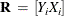and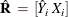The 2SLS estimator is defined as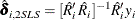Let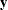and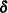be the vectors obtained by stacking the vectors of dependent variables and parameters for allequations, and letand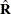be the block diagonal matrices formed by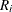and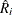, respectively.

The SUR and ITSUR estimators are defined as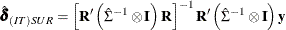while the 3SLS and IT3SLS estimators are defined as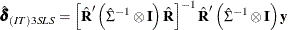whereis the identity matrix, and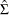is an estimator of the cross-equation correlation matrix. For 3SLS,is obtained from the 2SLS estimation, while for SUR it is derived from the OLS estimation. For IT3SLS and ITSUR, it is obtained iteratively from the previous estimation step, until convergence.

#### Computation of Standard Errors

The VARDEF= option in the PROC SYSLIN statement controls the denominator used in calculating the cross-equation covariance estimates and the parameter standard errors and covariances. The values of the VARDEF= option and the resulting denominator are as follows:

N

uses the number of nonmissing observations.

DF

uses the number of nonmissing observations less the degrees of freedom in the model.

WEIGHT

uses the sum of the observation weights given by the WEIGHTS statement.

WDF

uses the sum of the observation weights given by the WEIGHTS statement less the degrees of freedom in the model.

The VARDEF= option does not affect the model mean squared error, root mean squared error, or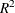statistics. These statistics are always based on the error degrees of freedom, regardless of the VARDEF= option. The VARDEF= option also does not affect the dependent variable coefficient of variation (CV).

#### Reduced Form Estimates

The REDUCED option in the PROC SYSLIN statement computes estimates of the reduced form coefficients. The REDUCED option requires that the equation system be square. If there are fewer models than endogenous variables, IDENTITY statements can be used to complete the equation system.

The reduced form coefficients are computed as follows. Represent the equation system, with all endogenous variables moved to the left-hand side of the equations and identities, as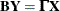Here B is the estimated coefficient matrix for the endogenous variables Y, and
is the estimated coefficient matrix for the exogenous (or predetermined) variables X.

The system can be solved for Y as follows, provided B is square and nonsingular: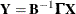The reduced form coefficients are the matrix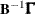.

#### Uncorrelated Errors across Equations

The SDIAG option in the PROC SYSLIN statement computes estimates by assuming uncorrelated errors across equations. As a result, when the SDIAG option is used, the 3SLS estimates are identical to 2SLS estimates, and the SUR estimates are the same as the OLS estimates.

#### Overidentification Restrictions

The OVERID option in the MODEL statement can be used to test for overidentifying restrictions on parameters of each equation. The null hypothesis is that the predetermined variables that do not appear in any equation have zero coefficients. The alternative hypothesis is that at least one of the assumed zero coefficients is nonzero. The test is approximate and rejects the null hypothesis too frequently for small sample sizes.

The formula for the test is given as follows. Let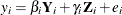be the i th equation.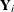are the endogenous variables that appear as regressors in the i th equation, and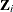are the instrumental variables that appear as regressors in the i th equation. Let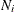be the number of variables inand.

Let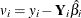. Let Z represent all instrumental variables, T be the total number of observations, and K be the total number of instrumental variables. Defineas follows: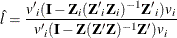Then the test statistic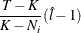is distributed approximately as an F with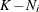and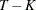degrees of freedom. See Basmann (1960) for more information.

#### Fuller’s Modification to LIML

The ALPHA= option in the PROC SYSLIN and MODEL statements parameterizes Fuller’s modification to LIML. This modification is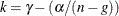, whereis the value of the ALPHA= option,is the LIML k value,n is the number of observations, and g is the number of predetermined variables. Fuller’s modification is not used unless the ALPHA= option is specified. See Fuller (1977) for more information.